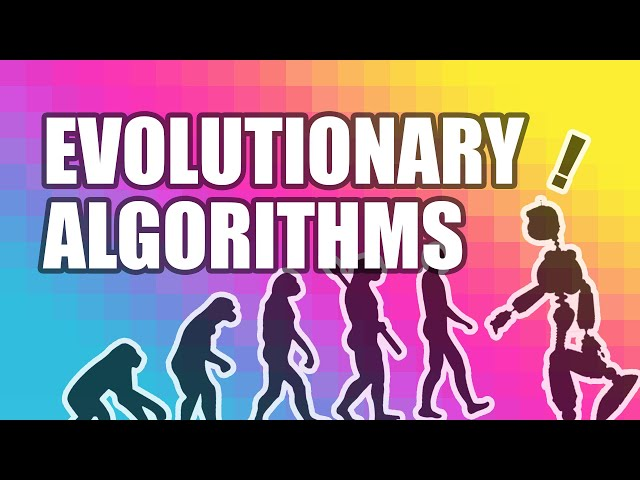# What is Evolutionary Algorithms

##### Introduction

In the world of computer science, an important area of research is the development of algorithms. However, sometimes, traditional algorithms are not enough to solve very complex problems. In such scenarios, a class of algorithms, known as evolutionary algorithms, can be very useful. These algorithms use evolutionary principles to search for optimal solutions to problems.

##### What are Evolutionary Algorithms?

Evolutionary algorithms are a class of search algorithms that use the principles of Darwinian evolution to search for optimal solutions in a given search space. Evolutionary algorithms work on the principle of natural selection, and they aim to find the best possible solution to a problem, just as natural selection aims to produce the fittest organisms.

##### How do Evolutionary Algorithms Work?

Evolutionary algorithms start with a population of possible solutions to a problem. These solutions are then evaluated against a fitness function, which defines how well the solution performs in solving the problem. Based on their fitness scores, some solutions are selected for reproduction, while others are discarded.

The solutions that are selected for reproduction undergo some changes, which are based on mutation or crossover. These changes create new offspring that are added to the population. The process of selection, mutation, and reproduction is repeated over multiple iterations, and it leads to the evolution of better and better solutions to the problem.

##### Types of Evolutionary Algorithms

There are several types of evolutionary algorithms, and some of the most common ones are:

• Genetic algorithms
• Evolution strategies
• Evolutionary programming
• Genetic programming

Genetic Algorithms

Genetic algorithms (GAs) are the most common type of evolutionary algorithms. They are used to solve optimization problems that involve searching for the minima or maxima of a function. GAs start with an initial population of candidate solutions, which are represented as strings of binary, integer, or real-valued values.

Each candidate solution is evaluated using a fitness function, and the solutions with the best fitness scores are selected for reproduction. The reproduction involves combining the genetic material of two or more selected solutions, and creating new offspring. Mutation and crossover are two mechanisms that are used for this purpose.

Mutation involves making a small, random change to a candidate solution, while crossover involves combining two or more candidate solutions to create a new, modified solution. The process of selection, mutation, and crossover is repeated over multiple generations, and it eventually leads to the evolution of better and better solutions to the problem.

Evolution Strategies

Evolution strategies (ES) are a family of optimization techniques that are similar to genetic algorithms. However, instead of using binary or real-valued representations, ES algorithms use real-valued vectors to represent solutions to a problem. An ES algorithm starts with an initial population of real-valued vectors, which are evaluated using an objective function.

The best vectors are selected for reproduction, and their genetic material is combined to create new offspring. However, unlike in GAs, there is no crossover involved in reproduction. Instead, the offspring are generated by adding small, random, normally-distributed values to the parent vectors. This process is called mutation. The offspring are then evaluated, and the best ones are selected as parents for the next generation.

Evolutionary Programming

Evolutionary programming (EP) is a subset of evolutionary algorithms that is used to solve optimization problems that involve continuous values. EP algorithms start with an initial population of randomly generated solutions, which are evaluated using the objective function.

The solutions with the best fitness scores are selected for reproduction, which involves adding a small, normally distributed random vector to the selected solutions. This process is similar to mutation. The offspring are then evaluated, and the best ones are selected as parents for the next generation.

The process of selection, mutation, and reproduction is repeated over multiple generations, and it eventually leads to the evolution of better and better solutions to the problem.

Genetic Programming

Genetic programming (GP) is a type of evolutionary algorithm that is used to evolve computer programs that solve a particular problem. GP starts with an initial population of random computer programs, which are represented as trees.

Each tree represents a computer program, where the root of the tree represents the main function, and the branches of the tree represent sub-functions. The leaves of the tree represent the input variables and constants.

The fitness of each program is evaluated using a fitness function, which assesses how well the program solves the given problem. The programs with the best fitness scores are selected for reproduction, which involves crossover and mutation. The offspring are then evaluated, and the best ones are selected as parents for the next generation.

##### Applications of Evolutionary Algorithms

Evolutionary algorithms have found applications in many areas of computer science and engineering. Some common areas of application include:

• Control engineering
• Robotics
• Signal processing
• Finance
• Design optimization

In control engineering, evolutionary algorithms are used to design controllers for complex systems, such as aircraft and spacecraft. In robotics, evolutionary algorithms are used to evolve robots that can perform specific tasks, such as walking or climbing.

In signal processing, evolutionary algorithms can be used to optimize the parameters of filters, such as the coefficients of an adaptive filter. In finance, evolutionary algorithms can be used to estimate the parameters of option pricing models, such as the Black-Scholes model.

In design optimization, evolutionary algorithms can be used to find the optimal design of a given system, such as the shape of an airplane wing or the layout of a circuit board.

##### Conclusion

Evolutionary algorithms are a powerful class of search algorithms that can be used to solve complex optimization problems. These algorithms use the principles of natural selection to guide the search for optimal solutions, and they have found applications in many areas of science and engineering. With the continued development of computer hardware and software, evolutionary algorithms are likely to become even more useful in the future.